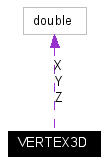﻿ VERTEX3D structure

VERTEX3D structure

Declared in: GeoTransformers.h

Single 3D point. Could be referring to a ground coordinate or any other 3D space (usually cartesian).

[C / C++]

typedef struct {

double X; // X-coordinate of a 3D point

double Y; // Y-coordinate of a 3D point

double Z; // Z-coordinate of a 3D point

} VERTEX2D;

Collaboration diagram:Collaboration graph for VERTEX3D structure

Members

X

X-coordinate of the 3D point. (we use X-axis for easting)

Y

Y-coordinate of the 3D point. (we use Y-axis for northing)

Z

Z-coordinate of the 3D point. (we use Z-axis for elevation)

Requirements

 Platform 32 bit Windows OS Environment ANSI C / C++ Standard compliant

Example

For a detailed example using this structure, please see Custom GeoTransformer VC++ Sample.

Related Topics

Referenced By: ARRAY3D, GeoTransData::GTD